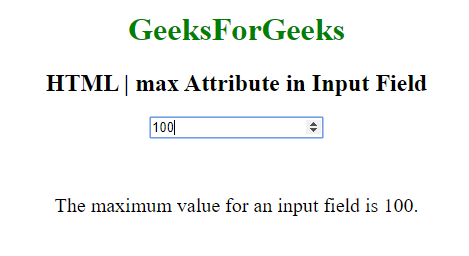Open in App
Not now

# HTML | max attribute

• Last Updated : 24 Aug, 2022

In HTML max attribute it specifies the maximum value of an element. when max attribute is used by progress element, it specifies how much work the task requires in the total task.

Applies to: The max attribute are used on the following elements:

Example:

## HTML

 ```<``html``>``<``body` `style="text-align:center;">``    ``<``h1` `style="color:green;">``        ``GeeksForGeeks``    ````    ``<``h2``>``        ``HTML | max Attribute in Input Field``    ````    ``<``form` `id="myGeeks">``        ``<``input` `type="number"``               ``id="myNumber"``               ``step="5"``               ``name="geeks"``               ``placeholder="multiples of 5"``               ``max="100">``    ````    ``<``br``>``    ``<``br``>``    ``<``p` `style="font-size:20px;">``        ``The maximum value for an input field is 100.``    ```````

Output:Supported Browsers: The browser supported by HTML | max Attribute are listed below:

• Google Chrome 4 and above
• Edge 12 and above
• Opera 12.1 and above
• Safari 5 and above
• Firefox 16 and above
My Personal Notes arrow_drop_up